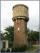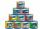# Find two

Find two consecutive natural numbers whose product is 1 larger than their sum. Searched numbers expressed by a fraction whose numerator is the difference between these numbers and the denominator is their sum.

Result

a =  2
b =  3

#### Solution:Checkout calculation with our calculator of quadratic equations.Leave us a comment of example and its solution (i.e. if it is still somewhat unclear...):

Showing 0 comments:Be the first to comment!#### To solve this example are needed these knowledge from mathematics:

Looking for help with calculating roots of a quadratic equation? Do you have a linear equation or system of equations and looking for its solution? Or do you have quadratic equation?

## Next similar examples:

1. Quadratic equationFind the roots of the quadratic equation: 3x2-4x + (-4) = 0.
2. VariableFind variable P: PP plus P x P plus P = 160
3. Expression with powersIf x-1/x=5, find the value of x4+1/x4
4. Basket of fruitIn six baskets, the seller has fruit. In individual baskets, there are only apples or just pears with the following number of fruits: 5,6,12,14,23 and 29. "If I sell this basket," the salesman thinks, "then I will have just as many apples as a pear." Which
5. Theorem proveWe want to prove the sentense: If the natural number n is divisible by six, then n is divisible by three. From what assumption we started?
6. Difference of two numberThe difference of two numbers is 20. They are positive integers greater than zero. The first number raised to one-half equals the second number. Determine the two numbers.
7. Water reservoirThe cuboid reservoir contains 1900 hectoliters of water and the water height is 2.5 m. Determine the dimensions of the bottom where one dimension is 3.2 m longer than the second one.
8. ChildrenThe group has 42 children. There are 4 more boys than girls. How many boys and girls are in the group?
9. EquationEquation ? has one root x1 = 8. Determine the coefficient b and the second root x2.
10. DiscriminantDetermine the discriminant of the equation: ?
11. Solve 3Solve quadratic equation: (6n+1) (4n-1) = 3n2
12. RootsDetermine the quadratic equation absolute coefficient q, that the equation has a real double root and the root x calculate: ?
13. FractionFor what x expression ? equals zero?
14. CansHow many cans must be put in the bottom row if we want 182 cans arrange in 13 rows above so that each subsequent row has always been one tin less? How many cans will be in the top row?
15. Quadratic inequationIf 5x + x² > 100, then x is not
16. CandiesIn the box are 12 candies that look the same. Three of them are filled with nougat, five by nuts, four by cream. At least how many candies must Ivan choose to satisfy itself that the selection of two with the same filling? ?
17. EquationHow many real roots has equation ? ?